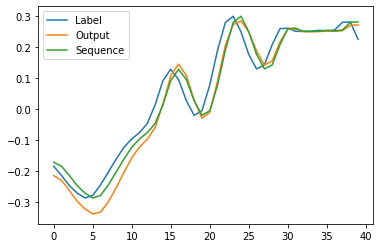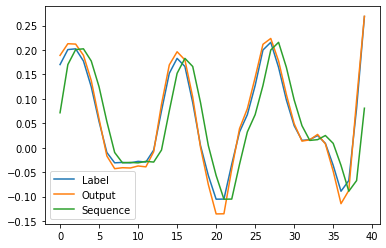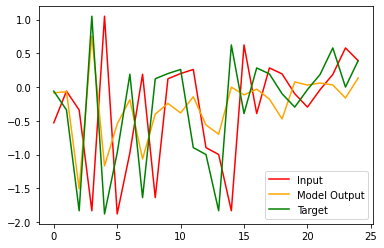# LSTM time series prediction network copies the input

I am creating a model for music generation, but my proble is that for some reason modelpredicts a current step, and not a next step as a label says, even though i compute loss between model output and labels, and labels are shifted by 1 forward:Sequence - model input
Label - sequence shifted 1 step forward
Output - model output

And if i feed in the label as input, it “predicts” the label, so model is basically repeating the inputDataset code:

``````class h5FileDataset(Dataset):
def __init__(self, h5dir, seq_length):
self.h5dir = h5dir
self.seq_length = seq_length + 1
with h5py.File(h5dir,'r') as datafile:
self.length = len(datafile['audio']) // self.seq_length
def __len__(self):
return self.length
def __getitem__(self,idx):
with h5py.File(self.h5dir,'r') as datafile:
seq = datafile["audio"][idx*self.seq_length:idx*self.seq_length+self.seq_length]

feature = seq[0:len(seq)-1].astype('float32') #from 0 to second-to last element
label = seq[1:len(seq)].astype('float32') #from 1 to last element

return feature,label
``````

Model code:

``````class old_network(nn.Module):
def __init__(self, input_size=1, hidden_layer_size=1, output_size=1, seq_length_ = 1, batch_size_ = 128):
super().__init__()
self.hidden_layer_size = hidden_layer_size
self.batch_size = batch_size_
self.seq_length = seq_length_

self.lstm = nn.LSTM(input_size, hidden_layer_size, batch_first = False, num_layers = 2)

self.linear1 = nn.Linear(hidden_layer_size, output_size)
self.linear2 = nn.Linear(hidden_layer_size, output_size)
#self.tanh1 = nn.Tanh()
self.tanh2 = nn.Tanh()

def forward(self, input_seq):
lstm_out, _ = self.lstm(input_seq)
lstm_out = lstm_out.reshape(lstm_out.size(1),lstm_out.size(0),1) #reshape to batch,seq,feature
predictions = self.linear1(lstm_out)
#predictions2 = self.tanh1(predictions1)
predictions = self.linear2(predictions)
predictions = self.tanh2(predictions)
return predictions.reshape(predictions.shape,predictions.shape,1) #reshape to seq,batch,feature to match labels shape
``````

Training loop:

``````epochs = 10
losses = [[],[]]
print("Starting training...")
try:
for epoch in range(epochs):
batch = 1
start = time.time()
seq = seq.reshape(seq_length,batch_size,1).to(DEVICE)
labels = labels.reshape(seq_length,batch_size,1).to(DEVICE)

y_pred = model(seq)

loss = loss_function(y_pred, labels)
loss.backward()
optimizer.step()

try:
eval_seq, eval_labels = next(eval_iter)
except StopIteration:
eval_seq, eval_labels = next(eval_iter)
eval_seq = eval_seq.reshape(seq_length,batch_size,1).to(DEVICE)
eval_labels = eval_labels.reshape(seq_length,batch_size,1).to(DEVICE)

eval_y_pred = model(eval_seq)

eval_loss = loss_function(eval_y_pred, eval_labels)
losses.append(eval_loss.item())
losses.append(loss.item())

print_inline("Batch {}/{} Time/batch: {:.4f}, Loss: {:.4f} Loss_eval: {:.4f}".format(batch,batches,time.time()-start, loss.item(), eval_loss.item()))
batch += 1

if batch%50 == 0:
print("\n Epoch: {}/{} Batch:{} Loss_train:{:.4f} Loss_eval: {:.4f}".format(epoch,epochs,batch,loss.item(),eval_loss.item()))

plt.close()
plt.plot(range(0,len(losses)),losses, label = "Learning dataset")
plt.plot(range(0,len(losses)),losses, label = "Evaluation dataset")
plt.legend()
plt.show()
torch.save({'model_state_dict':model.state_dict(), 'optimizer_state_dict' : optimizer.state_dict()},save_dir)

except KeyboardInterrupt:
plt.close()
plt.plot(range(0,len(losses)),losses, label = "Learning dataset")
plt.plot(range(0,len(losses)),losses, label = "Evaluation dataset")
plt.legend()
plt.show()
``````

I am kinda running out of ideas by this point, not sure what is wrong

No real ideas, just some comments:

(1) I would never use `reshape()` or `view()` to adjust the tensor shape. I’ve seen to many cases where this was used incorrectly and broke the tensor. Just because the shape is correct in the network doesn’t throw an error doesn’t mean the tensor is correct. If possible, I always use `transpose()` or `permute()` since in almost all cases I only need to swap dimensions. For exanole, instead of

``````seq = seq.reshape(seq_length,batch_size,1).to(DEVICE)
``````

I would do

``````seq = seq.tranpose(1,0).to(DEVICE)
``````

or

``````seq = seq.permute(1,0,2).to(DEVICE)
``````

This ensures that dimensions are only swapped but never “torn apart” which can happen with `reshape()` or `view()`. The latter are mostly needed to maybe (un-)flatten tensors, but that’s not needed here.

(2) I’m also not quite sure about

``````predictions = self.linear1(lstm_out)
``````

since the shape of `lstm_out` is `(batch_size, seq_len, features)`. I know that `nn.Linear` takes as input `(N,∗,H_in)` but I’m not sure if you really want go that way. Usually the last hidden state is used for prediction. So I would try:

``````lstm_out, (h, c) = self.lstm(input_seq)
predictions = self.linear1(h[-1])
``````

`h[-1]` is the last layer of the last hidden state.

This is a classic result of using LSTM for time series analysis. LSTM is simply using the hidden state to relay back an earlier input without actually learning any patterns. In order to trick the LSTM into learning patterns, you can do the following

• Reduce step size
• Increase HiddenDim size
``````%matplotlib inline
import matplotlib.pyplot as plt

import torch
import torch.nn as nn
import torch.nn.functional as F
import torch.optim as optim
import numpy as np

class LSTMSimple(nn.Module):
def __init__(self,inputDim,hiddenDim,batchSize,outputDim):
super(LSTMSimple,self).__init__()
torch.manual_seed(1)
self.lstm=nn.LSTM(inputDim,hiddenDim,1).cuda()
# Hidden state is a tuple of two states, so we will have to initialize two tuples
self.state_h = torch.randn(1,batchSize,hiddenDim).cuda()
self.state_c = torch.rand(1,batchSize,hiddenDim).cuda()
self.linearModel=nn.Linear(hiddenDim,outputDim).cuda()

def forward(self,inputs):
# LSTM
output, self.hidden = self.lstm(inputs, (self.state_h,self.state_c) )
self.state_h=self.state_h.detach()
self.state_c=self.state_c.detach()
# LINEAR MODEL
output=self.linearModel(output).cuda()
return output

def lossCalc(x,y):

# Model Object
batchSize=5
inputDim=1
outputDim=1
stepSize=5
hiddenDim=20
model=LSTMSimple(inputDim,hiddenDim,batchSize,outputDim).cuda()
loss = torch.nn.MSELoss()

# Input Data
dataInput = np.random.randn(stepSize*batchSize,inputDim)
dataY=np.insert(dataInput[1:],len(dataInput)-2,0)
dataInput=Variable(torch.from_numpy(dataInput.reshape(stepSize,batchSize,inputDim).astype(np.float32))).cuda()
dataY=Variable(torch.from_numpy(dataY.reshape(stepSize,batchSize,inputDim).astype(np.float32))).cuda()
for epoch in range(10000):
dataOutput=model(dataInput).cuda()
curLoss=loss(dataOutput.view(batchSize*stepSize,outputDim),dataY.view(batchSize*stepSize,outputDim))
curLoss.backward()
optimizer.step()
if(epoch % 1000==0):
print("For epoch {}, the loss is {}".format(epoch,curLoss))

plt.plot(dataInput.cpu().detach().numpy().reshape(-1),color="red")
plt.plot(dataOutput.cpu().detach().numpy().reshape(-1),color="orange")
plt.plot(dataY.cpu().detach().numpy().reshape(-1),color="green")
plt.figure()
``````What do you mean exactly by reducing step size? Reducing input and output sequence lengths?

Tried increasing hidden dim to 100 and reducing seq_length to 250, still follows the input
Loss graph:Seq Len 250 is still very high for LSTM. Can you reduce it to 3 or 5 and retry

Oh, i thought that lstm needs long sequences, especially in things like music, to capture all long-term dependencies